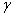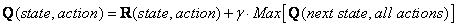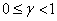## Q-Learning Algorithm

Our virtual agent will learn through experience without teacher (this is called unsupervised learning). The agent will explore state to state until it reaches the goal. We call each exploration as an episode . In one episode the agent will move from initial state until the goal state. Once the agent arrives at the goal state, program goes to the next episode. The algorithm below has been proved to be convergence (See references for the proof )

Q Learning

Given : State diagram with a goal state (represented by matrix R )

Find : Minimum path from any initial state to the goal state (represented by matrix Q )

 Q Learning Algorithm goes as follow Set parameter, and environment reward matrix R Initialize matrix Q as zero matrix For each episode: Select random initial state Do while not reach goal state Select one among all possible actions for the current state Using this possible action, consider to go to the next state Get maximum Q value of this next state based on all possible actions ComputeSet the next state as the current state End Do End For

The above algorithm is used by the agent to learn from experience or training. Each episode is equivalent to one training session. In each training session, the agent explores the environment (represented by Matrix R ), get the reward (or none) until it reach the goal state. The purpose of the training is to enhance the 'brain' of our agent that represented by Q matrix. More training will give better Q matrix that can be used by the agent to move in optimal way. In this case, if the Q matrix has been enhanced, instead of exploring around and go back and forth to the same room, the agent will find the fastest route to the goal state.

Parameterhas range value of 0 to 1(). Ifis closer to zero, the agent will tend to consider only immediate reward. Ifis closer to one, the agent will consider future reward with greater weight, willing to delay the reward.

To use the Q matrix, the agent traces the sequence of states, from the initial state until goal state. The algorithm is as simple as finding action that makes maximum Q for current state:

 Algorithm to utilize the Q matrix Input: Q matrix, initial state Set current state = initial state From current state, find action that produce maximum Q value Set current state = next state Go to 2 until current state = goal state

The algorithm above will return sequence of current state from initial state until goal state.